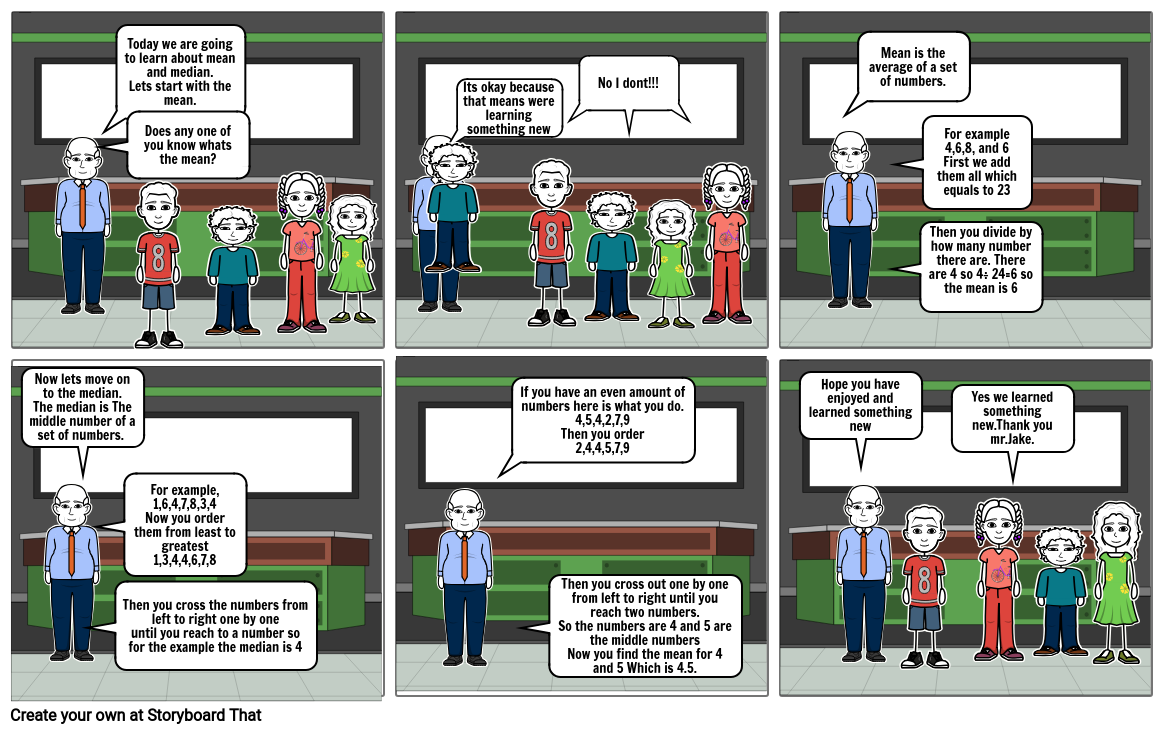# Math Mean and MedianMore Options: Make a Folding Card

#### Storyboard Description

This storyboard does not have a description.

#### Storyboard Text

• Now lets move on to the median.The median is when there is a set of numbers and
• Does any one of you know whats the mean?
• Its okay because that means were learning something new
• No I dont!!!
• Mean is the average of a set of numbers.
• Then you divide by how many number there are. There are 4 so 4÷ 24=6 so the mean is 6
• For example4,6,8, and 6First we add them all which equals to 23
• Now lets move on to the median.The median is The middle number of a set of numbers.
• Then you cross the numbers from left to right one by oneuntil you reach to a number so for the example the median is 4
• For example,1,6,4,7,8,3,4Now you order them from least to greatest1,3,4,4,6,7,8
• If you have an even amount of numbers here is what you do.4,5,4,2,7,9Then you order2,4,4,5,7,9
• Then you cross out one by one from left to right until you reach two numbers.So the numbers are 4 and 5 are the middle numbersNow you find the mean for 4 and 5 Which is 4.5.
• Hope you have enjoyed and learned something new
• Yes we learned something new.Thank you mr.Jake.
More Storyboards By b1952590
Explore Our Articles and Examples

### Teacher Resources

Lesson Plans Worksheet TemplatesPoster TemplatesStoryboard Templates